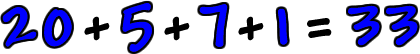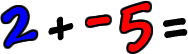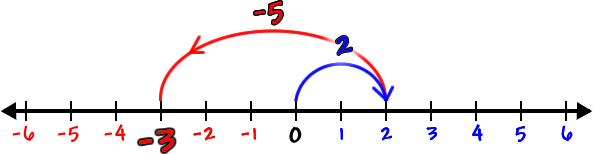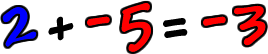I'm going to use number lines and my little math car a lot in this lesson.  It's really the only good way to get this into your head!  So, if you didn't see the last lesson, please check it out now.

Before, we did addition when all the numbers had the same sign.  Here's a quick review:

If all the numbers are positive, then the answer is positive.If all the numbers are negative, then the answer is negative.Note:  The easy way to do this problem is to just ignore all the negative signs, add them up like positive numbers, then stick a negative sign on your answer!

OK, we're cool with that...

So... What if some of the numbers are positive and some are negative?

Will the answer be positive or negative?

It all depends on the numbers!

Let's do some with our car and the number lines and see how it works:

* Remember, when the number is positive, the car drives forward and, when the number is negative, the car backs up.press the purple "play" button to start the car

Here's it is on the number line: